# Mass and co-mass

Adjoint norms (cf. Norm) in certain vector spaces dual to each other.

1) The mass of an-vector, i.e. an element of the-fold exterior product of a vector space, is the numberThe co-mass of an-covectoris the number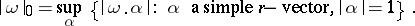Here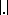is the standard norm of an-vector and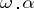is the scalar product of a vector and a covector.

The mass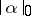and the co-mass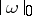are adjoint norms in the spaces of-vectors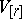and-covectors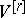, respectively. In this connection:

a),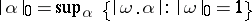;

b),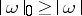, and equalities hold if and only if() is a simple-(co)vector;

c)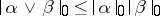,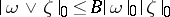for exterior products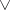, where for a simple multi-covector(or)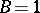, and, in general,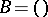if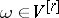and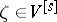;

d)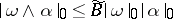for inner products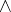, where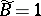for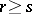and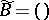for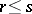,and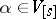.

These definitions enable one to define the mass and co-mass for sections of fibre bundles whose standard fibres areand. For example, the co-mass of a formon a domain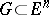is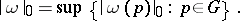2) The mass of a polyhedral chainis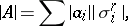where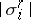is the volume of the cell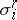. For arbitrary chains the mass (finite or infinite) can be defined in various ways; for flat chains (see Flat norm) and sharp chains (see Sharp norm) these give the same value to the mass.

3) The co-mass of a (flat, in particular, sharp) cochainis defined in the standard way:whereis a polyhedral chain and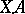is the value of the cochainon the chain.

For references see Flat norm.

A simple-vectoris an element of the form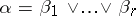in the-fold exterior productof a vector space. Here "" denotes exterior product and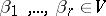.